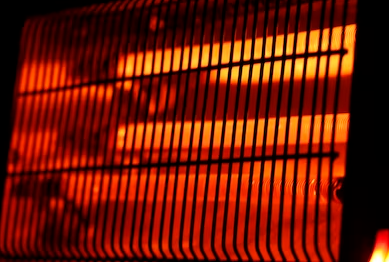## Current

Consider an electric space heater is built with a 40 ohm resistor. How much current (amps) is needed to produce 500 W of heat?Expand Hint
Power absorbed by a resistive element:
$$P=VI=\frac{V^{2}}{R}=I^2R$$$where $$V$$ is voltage, $$I$$ is current, and $$R$$ is resistance. Hint 2 Based on the problem statement’s givens, solve for current using the equation: $$P=I^2R$$$
Power absorbed by a resistive element:
$$P=VI=\frac{V^{2}}{R}=I^2R$$$where $$V$$ is voltage, $$I$$ is current, and $$R$$ is resistance. Solving for Current using the problem statement’s knowns: $$P=I^2R\to 500W=I^2(40\Omega)$$$
$$I=\sqrt{\frac{500W}{40\Omega}}=3.5\:A$$\$
3.5 A
Similar Problems from FE Sub Section: Power Absorbed by a Resistive Element

Similar Problems from FE Section: Electrostatics